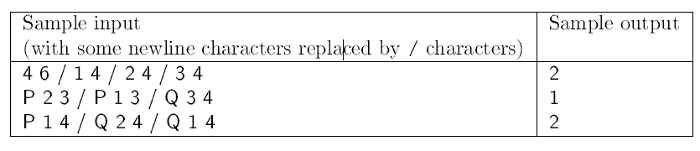Mathematical and Physical Journal
for High Schools
Issued by the MATFUND Foundation
 Already signed up? New to KöMaL?

#Problem S. 108. (May 2016)

S. 108. Andrew and Brian play a certain game on a tree graph having $\displaystyle N$ ($\displaystyle 1\le N\le 100\,000$) vertices. The game consists of $\displaystyle M$ ($\displaystyle 1\le M\le 100\,000$) steps. Each step is one of the following two possibilities.

– Andrew selects two vertices of the tree, and puts one chocolate on every edge of the unique path between the vertices.

– Brian asks for the number of chocolates on a given edge.

The goal is to answer Brian's questions as quickly as possible.

Your program should read the values of $\displaystyle N$ and $\displaystyle M$ from the first line of the standard input, then the tree edges (represented by the integers $\displaystyle u$ and $\displaystyle v$) from the following $\displaystyle N-1$ lines. Then each of the following $\displaystyle M$ lines starts with either a letter P or Q, followed by a pair of integers $\displaystyle u, v$. This last pair denotes a pair of vertices. Letter P'' means that Andrew has put one chocolate on every edge of the unique path between the vertices $\displaystyle (u, v)$. Letter Q'' means that Brian has asked for the number of chocolates on the edge $\displaystyle (u, v)$. Your program should write the answer to Brian's questions in the successive lines of the standard output.Scoring and bounds. You can get 1 point for a brief and proper documentation clearly describing your solution. Nine further points can be obtained provided that your program solves any valid input within 1 second of running time.

The source code of your program with a short documentation—also describing which developer environment to use for compiling the source—should be submitted in a compressed file s108.zip.

(10 pont)

Deadline expired on June 10, 2016.

### Statistics:

 8 students sent a solution. 10 points: Gáspár Attila. 9 points: Janzer Orsolya Lili, Németh 123 Balázs, Noszály Áron. 8 points: 2 students. 3 points: 1 student. 1 point: 1 student.

Problems in Information Technology of KöMaL, May 2016